##1. Isotomic image of a line

Here I continue the discussion started in the file BarycentricCoordinates3.html . There we saw that given a point P0(x0,y0,z0), the conic (c0) given by equation
x0*y*z + y0*z*x + z0*x*y = 0, is generated in two ways:
- Through the tripols tr(L) of all lines L through a fixed point P0.
- Through the isotomic conjugates t(Q) of all points Q on the dual line ~P0 of P0.

The two ways are related: If QL = L*(~P0) is the intersection of L with the dual of P0, then {tr(L), t(P0), t(QL)} are collinear.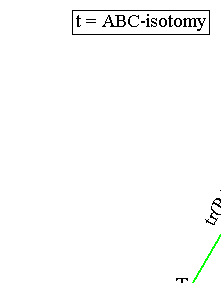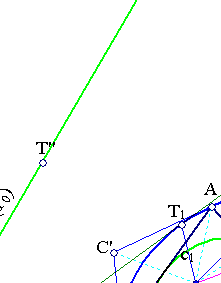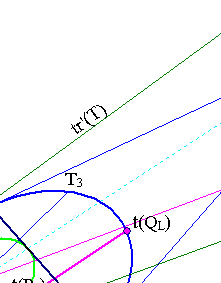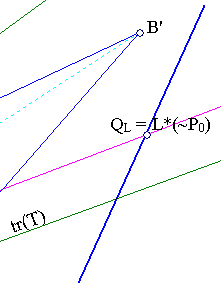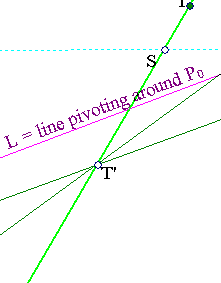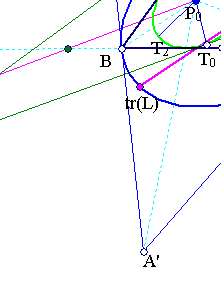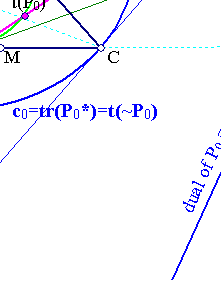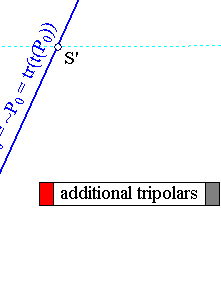In fact the asserted collinearity is equivalent with the vanishing of the determinant with rows:
(1/x0, 1/y0, 1/z0) (point t(P0))
(1/a, 1/b, 1/c) (point tr(L))
(1/(bz0-cy0), 1/(cx0-az0), 1/(ay0-bx0)) (point t(QL)).
Here ax+by+cz=0 denotes the variable line L through (x0,y0,z0), hence satisfying ax0+by0+cz0=0.
Through an easy calculation the vanishing of this determinant reduces exactly to this last equation.
More relations between various lines and the conics related to to isogonal points {P0, t(P0)} are displayed in IsotomicChart.html .

##2. Remarks on the various tripolars

Here are some complementary remarks on the tripolars of points on the tripolar tr(P0) proved in IsogonalGeneralized.html .
 For every point T on tr(P0) the tripolar tr(T) is a tangent of the conic (c1) which is tangent to the sides of ABC at the traces of the cevians from P0. This is the dual conic of the previous one. The matrices of the two conics are inverse up to a multiplication factor. P0 is again called the perspector of c1 and tr(P0) the perspectrix of c1.
 tr(P0) is the polar of P0 with respect to both conics c0 and c1. Lines tr(P0) and ~P0 are isotomic conjugate in the sense that their intersection points with the sides of ABC are correspondingly symmetric with respect to the middle of the respective side (see (5) in IsogonalGeneralized.html ).
 For every point T on tr(P0) the tripolar tr'(T) with respect to the tangential triangle A'B'C' is tangent to c0.
 Lines tr(T) and tr'(T) intersect at a point T' on tr(P0).
 The contact points T0 = tr(T)*c1 and T1 = tr'(T)*c0 are collinear with P0. Line T0T1 is the polar of T' with respect to both conics c0 and c1.
 Analogously the tripolars of T', lines tr(T') and tr'(T') intersect at a point T''. Denote the contact points T2 = tr(T')*c1 and T3 = tr'(T')*c0. Then lines T0T2 and T1T3 intersect at T.

##3. Generalizing to arbitrary conjugations

The pair of conics (c0) and (c1) can be defined directly from the triangle without using the device of trilinear polar or isotomy or any other kind of conjugation, as exposed in IsogonalGeneralized.html . The two conics depend only on the triangle and the point P0 which determine immediately all the necessary data to a direct definition of them. In fact for the circumconic (c0) one needs the tangents at the vertices or the tangential triangle whose vertices are the harmonic associates of P0. Analogously the inconic (c1) needs the contact points with the sides of ABC which are the traces of P0.
In IsogonalGeneralized.html it is discussed a generalization of the isotomy (as well as isogonality) based on the selection of an arbitrary point K instead of the centroid G defining the barycentric coordinates. For each such system the corresponding conjugation is defined and the remarks made above are also valid. One has only to interpret the resulting duality in the right context.

##4. Another aspect of the same figure

The figure below represents another aspect of the one considered above. In this (tK) is the generalized conjugation defined in the way discussed in IsogonalGeneralized.html . It demonstrates the property of the duals ~P of points P on the trilinear polar tr(K). They pass all through K.
The direct proof using generalized trilinears with respect to the projective base {A,B,C,K} is trivial:
- K has coordinates (1,1,1).
- tr(K) has equation x+y+z=0.
- a point P(x0,y0,z0) on tr(K) satisfies the previous equation and its dual line ~P is x0x+y0y+z0z=0.
Hence passes through (1,1,1).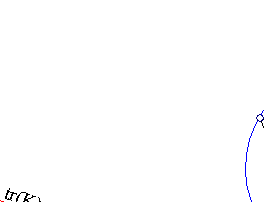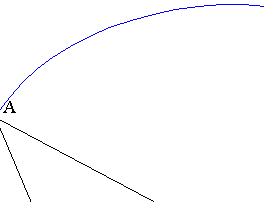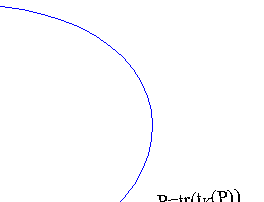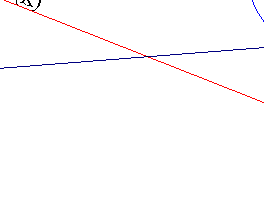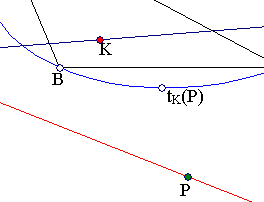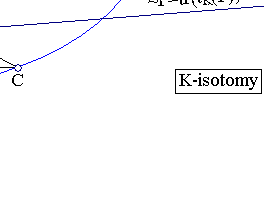##5. Particular cases

 For P0=K=G (G the centroid of ABC) the corresponding isotomy is the usual one (t) for which t(G)=G. Lines tr(G) and ~G coincide with the line at infinity. Conics c0, c1 are correspondingly the outer- inner- Steiner ellipses of the triangle.
 For P0 = K = the symmedian point of ABC, conics c0 and c1 are correspondingly the circumcircle and the Brocard ellipse of the triangle. Thus, we get the circumcircle of the triangle of reference, given (see CircumcircleInTrilinears.html ) in barycentrics by:
a2*y*z + b2*z*x + c2*x*y = 0,
as the image under t of the line a2*x + b2*y + c2*z, which is the trilinear polar of the triangle center X(76) (third Brocard Point) with barycentric coordinates (1/a2, 1/b2, 1/c2). A consequence is that the trilinear polar of every point on the circumcircle passes through the symmedian point of the triangle. Also, since the trilinear polar of K is the Lemoine axis, every point of this axis has trilinear polar a tangent to the circumcircle.